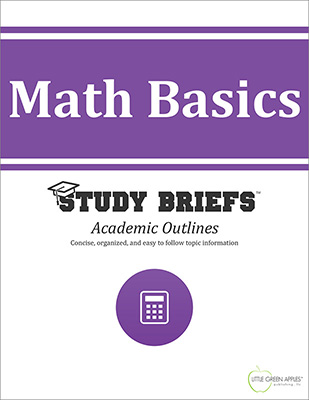# Math BasicsLook inside
• Format: eBook
• Edition: 2016
• Publisher: Little Green Apples Publishing LLC

Price: \$4.99

## Description:

Faced with an unexpected problem to solve that involves math, do you find yourself staring blankly at your calculator uncertain how to proceed or freezing up in a panic-stricken dread like a deer caught in headlights? This informative short read will help you recall with ease the common core set of basic math skills everyone is expected to know. According to mathematician, Stanley Gudder, "the essence of mathematics is not to make simple things commplicated, but to make complicated things simple." Our Math Basics Study Brief intends to do just that! This simple reference guide is intended as a straightforward and understandable review of basic math essentials. This academic outline provides a clear and concise conceptual overview with key terms and definitions highlighted and plenty of instructive examples stepped out in an easy to follow format. It is ideal for the school-age student who needs a refresher when moving from general math to college prep curriculum. Readers who suffer from math anxiety will discover an appealing and fact-filled introduction. It is also recommended as a convenient resource for lifelong learners interested in a brush-up of their knowledge of basic math topics. This Study Brief emphasizes basic math operations and practical methods of computation that are needed to achieve a solid foundation of comprehension before moving on to more advanced math concepts. Individual sections cover the number line, place value, rounding, math symbols, mathematical operators (addition, subtraction, multiplication, and division), fractions, ratios and proportions, conversion factors, exponents, roots, logarithms, as well as the basics of geometry, algebra, and probability and statistics.

## Topics Covered:

• Number Line
• Place Value
• Math Symbols
• Mathematical Operations
• Addition
• Subtraction
• Multiplication
• Division
• Fractions
• Ratios and Proportions
• Conversion Factors
• Exponents Roots and Logarithms
• Basics of Probability and Statistics
• Basic Geometry
• Basic Algebra
• References

Browse Subjects